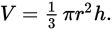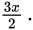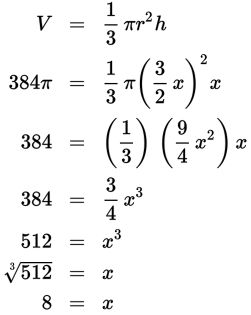# SAT Math Multiple Choice Question 570: Answer and Explanation

### Test Information

Question: 570

15. If a right cone is three times as wide at its base as it is tall, and the volume of the cone is 384π cubic inches, what is the diameter in inches of the base of the cone?

• A. 8
• B. 12
• C. 16
• D. 24

Explanation:

D

Difficulty: Hard

Category: Additional Topics in Math / Geometry

Strategic Advice: Don't forget to check the formulas provided for you at the beginning of each math section. The volume of a right cone is given byHere, you only know the value of one of the variables, V, so you'll need to use the information in the question to somehow write r and h in terms of just one variable.

Getting to the Answer: If the cone is three times as wide at the base as it is tall, then call the diameter 3x and the height of the cone one-third of that, or x. The volume formula calls for the radius, which is half the diameter, orSubstitute these values into the formula and solve for x:The question asks for the diameter of the base, which is 3x = 3(8) = 24.